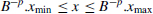## 3.3 REAL NUMBERS

As regards the real numbers, there are two types of approximations: fixed-point and floating-point numeration systems. The fixed-point system is a simple extension of the integer representation system; it allows the representation of a relatively reduced range of numbers with some constant absolute precision. The floating point system allows the representation of a very large range of numbers, with some constant relative precision.

Definitions 3.9

1. In a fixed-point numeration system, the number represented in the formis x/Bp, where x is the integer represented by the same sequence of digits without point.

2. Let xmin and xmax be the minimum and maximum integers that can be represented with n digits, that is, xmin = 1 − Bn−1 and xmax = Bn−1 − 1 in sign-magnitude representation, and xmin = − Bn/2 and xmax = Bn/2 − 1 in B's complement or excess-Bn/2 representation. Then, any real number x belonging to the intervalcan be represented in the form (3.21) with some error equal to the absolute value of the difference between x and its representation.

3. The distance d between exactly represented numbers is equal to the unit in the least significant position (ulp), that is, B−p, so that the maximum error is equal to
4. The maximum relative error is equal to then so that the maximum ...

Get Synthesis of Arithmetic Circuits: FPGA, ASIC and Embedded Systems now with the O’Reilly learning platform.

O’Reilly members experience books, live events, courses curated by job role, and more from O’Reilly and nearly 200 top publishers.